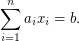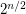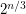# Exponential Algorithms for Knapsack

 Importance: Medium ✭✭
 Author(s): Lipton, Dick
 Subject: Theoretical Computer Science » Algorithms
 Keywords: Algorithm construction Exponential-time algorithm Knapsack
 Posted by: dick lipton on: February 4th, 2009
Conjecture

The famous 0-1 Knapsack problem is: Givenandintegers, determine whether or not there arevaluesso thatThe best known worst-case algorithm runs in timetimes a polynomial in. Is there an algorithm that runs in time?

## Bibliography

[SS] Richard Schroeppel and Adi Shamir. A T=O(2n/2), S=O(2n/4) algorithm for certain NP-complete problems, SIAM J. Comput. 10 (1981), no. 3, 456--464.

* indicates original appearance(s) of problem.

### References

Solving this problem in pseudopolynomial time with polynomial space: Daniel Lokshtanov and Jesper Nederlof: "Saving Space by Algebraization". To appear in the proceedings of ACM Symposium on Theory of Computing (STOC 2010).

The next reference solves this problem (even general knapsack, I believe). An 2^{0.311 n} Algorithm: Nick Howgrave-Graham and Antoine Joux : "A new generic algorithm for hard knapsacks". To appear in the proceedings of EUROCRYPT 2010.

However, the problem might be easily adjusted to "find an algorithm that takes O(2^{c n}) time, where c < 0.311", etc etc.

Publications from 2010 on this topic: Pseudopolynomial algorithm with only polynomial space: Daniel Lokshtanov and Jesper Nederlof: "Saving Space by Algebraization". To appear in the proceedings of ACM Symposium on Theory of Computing (STOC 2010).

Fast knapsack: Nick Howgrave-Graham and Antoine Joux: "New Generic Algorithms for Hard Knapsacks". To appear in the proceedings of EUROCRYPT 2010 The running time is O^*(2^0.311 n), which is faster than the question posted here.

Obviously, the question could become: find a lower constant than 0.311.

### 0-1 knapsack

I do not know how to determine the run time but I have an algorithm based on dynamic programming which solves the problem more efficiently than a simple search but not in polynomial time!

I have implemented the procedure as a Java program and tried to express it as a flow chart. Files for both of these are available at; www.cybase.co.uk/wlcs/Software.html

although at the moment the website is down.

I have contacted the ISP to try and restore it.

### This appears to be answered

see paper at http://www.joux.biz/publications/Knapsacks.pdf and rjlipton's blog post about it at http://rjlipton.wordpress.com/2010/02/05/a-2010-algorithm-for-the-knapsack-problem/

of course, this is an open ended question, there is still room for better algorithms.

### A solution has been announced

A (randomized, probably heuristic) algorithm has been announced to solve this problem in time close to 2^(0.311 n), at the ESC 2010 seminar.

For details, see: https://cryptolux.org/ESC/Antoine_Joux

### Subset sum or 0-1?

When your values - your a_n - can be negative, and your b (the goal) is zero, then it's called "subset sum". If the a_n are non-negative (i.e., some of the a's may be zero), the b is positive, and the choice is to either exclude (0) or include (1) one "copy" of each value, then it's a "0-1 knapsack" problem. Usually, but not always, a knapsack problem has components with multiple values, and the goal is a minimax problem: maximize the a's, while minimizing the c's.

It's always darkest, just after the lights go out.

### 0-1 Knapsack?

I know this problem as subset sum and not as 0-1 knapsack.